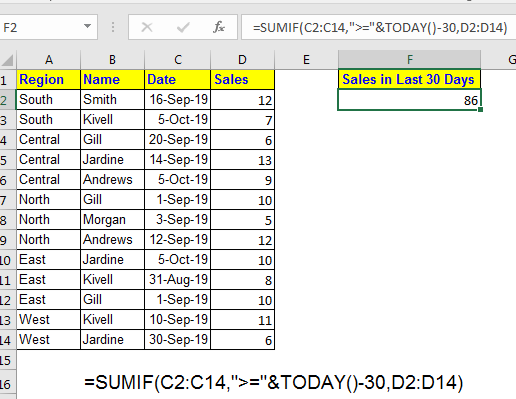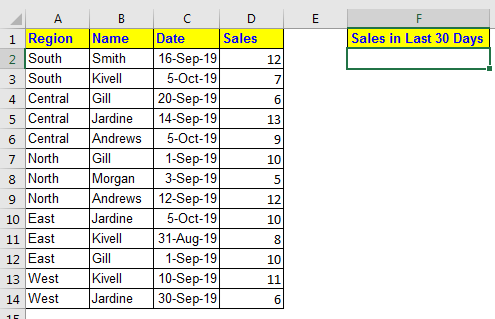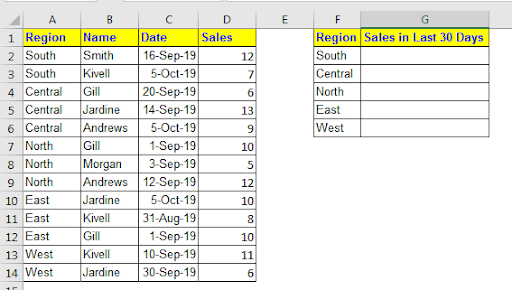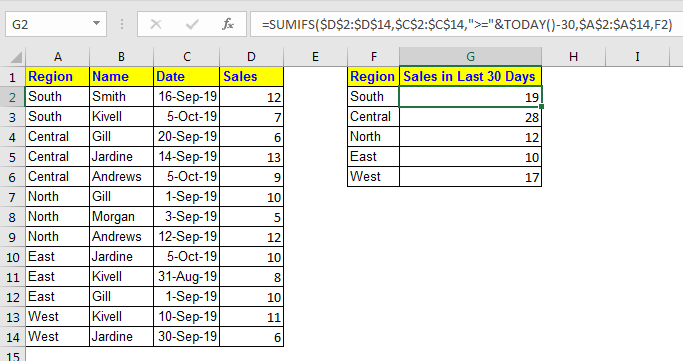# How to Sum Last 30 Days Sales in ExcelIn most of the industries, the sales and other values are summarised on monthly basis. Some times it is also summarized on standard 30 day interval. In that case, having a formula that dynamically shows the sum of last 30 days will be a good idea. In this article, we will learn how to sum last 30 days values in excel.

Generic Formula

=SUMIF(dates,">="&TODAY()-30, sum range)

Dates: this is the criteria range. It must contain the dates.
Sum range: this contains the values you want to sum.

Let’s see an example to make things clear.
Example: Sum Last 30 days Sales.
Here I have this data of sale done by employees of our company in last few months. Everyday when I open this sheet, I want to get a dynamic sum that shows me the total sales done in last 30 days.Apply above generic formula here to

=SUMIF(C2:C14,">="&TODAY()-30,D2:D14)

This will return the dynamic sum of last 30 days sales.How it works?

Well, it is quite simple. We simply used SUMIF function to some on a criteria. Let’s break this formula and see whats happening.

We have criteria range “C2:C14”.

And Criteria is ">="&TODAY()-30. It means, we want to sum any value that is greater than or equal to TODAY()-30. The TODAY function returns the current date. And we are subtracting 30 from it. Hence the start date for summing value, will not be before 30 days.

At last we have summed up the sales range D2:D14.

Sum Last 30 Days Sales in Different Categories
If you want the sum in categorised way, then its better to use SUMIFS function.Like, if you wanted to get sum of total sales in 30 days in different regions then the below formula would be perfect. Write this formula in G2 and copy it down. I have use Absolute Reference so that it doesn’t change when you copy formula in other cells.

=SUMIFS(\$D\$2:\$D\$14,\$C\$2:\$C\$14,">="&TODAY()-30,\$A\$2:\$A\$14,F2)This formula works same as the first one. It just, we have added one more criteria for region (\$A\$2:\$A\$14,F2). To do so, we use SUMIFS function. In SUMIFS function, the sum range is provided first, then the pairs of criteria range and criteria is provide. Unlike SUMIF, in SUMIFS you can have multiple criterias. You should read about it SUMIFS function.

So yeah guys, this how can sum values for last 30 days dynamically in excel. Not only 30 days, you can set years, months, weeks, anything. This can help you make dynamic dashboards for monitoring data. Let me know if you have any doubts regarding this article or any other excel/vba topic.
Related Articles:

SUMIFS using AND-OR logic

How to Use SUMIF Function in Excel

How to Use SUMIFS Function in Excel

Sum if cells contain specific text

Sum If Greater Than 0 in Excel

SUMIFS with dates in Excel

Popular Articles:
The VLOOKUP Function in Excel

COUNTIF in Excel 2016

How to Use SUMIF Function in Excel

Terms and Conditions of use

The applications/code on this site are distributed as is and without warranties or liability. In no event shall the owner of the copyrights, or the authors of the applications/code be liable for any loss of profit, any problems or any damage resulting from the use or evaluation of the applications/code.